## Finding Common Differences

The values of the truck in the example are said to form an arithmetic sequence because they change by a constant amount each year. Each term increases or decreases by the same constant value called the common difference of the sequence. For this sequence, the common difference is –3,400.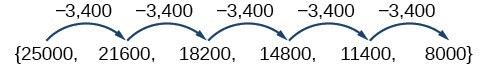The sequence below is another example of an arithmetic sequence. In this case, the constant difference is 3. You can choose any term of the sequence, and add 3 to find the subsequent term.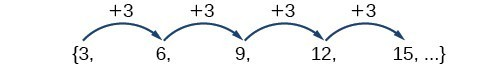### A General Note: Arithmetic Sequence

An arithmetic sequence is a sequence that has the property that the difference between any two consecutive terms is a constant. This constant is called the common difference. If ${a}_{1}$ is the first term of an arithmetic sequence and $d$ is the common difference, the sequence will be:

$\left\{{a}_{n}\right\}=\left\{{a}_{1},{a}_{1}+d,{a}_{1}+2d,{a}_{1}+3d,…\right\}$

### Example 1: Finding Common Differences

Is each sequence arithmetic? If so, find the common difference.

1. $\left\{1,2,4,8,16,…\right\}$
2. $\left\{-3,1,5,9,13,…\right\}$

### Solution

Subtract each term from the subsequent term to determine whether a common difference exists.

1. The sequence is not arithmetic because there is no common difference.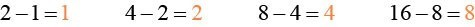2. The sequence is arithmetic because there is a common difference. The common difference is 4.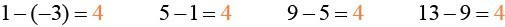### Analysis of the Solution

The graph of each of these sequences is shown in Figure 1. We can see from the graphs that, although both sequences show growth, $a$ is not linear whereas $b$ is linear. Arithmetic sequences have a constant rate of change so their graphs will always be points on a line.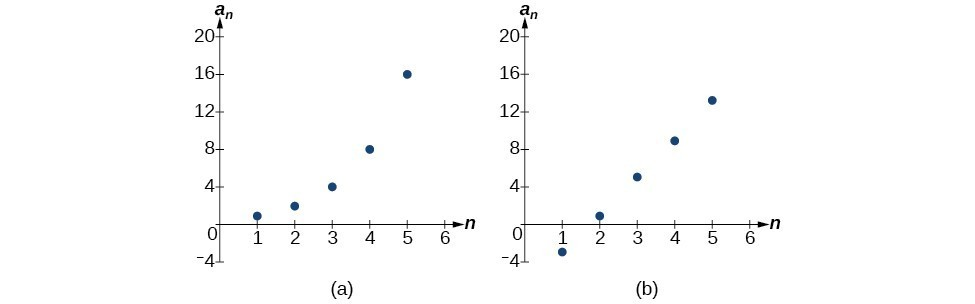Figure 1

### If we are told that a sequence is arithmetic, do we have to subtract every term from the following term to find the common difference?

No. If we know that the sequence is arithmetic, we can choose any one term in the sequence, and subtract it from the subsequent term to find the common difference.

### Try It 1

Is the given sequence arithmetic? If so, find the common difference.

$\left\{18,\text{ }16,\text{ }14,\text{ }12,\text{ }10,\dots \right\}$

### Try It 2

Is the given sequence arithmetic? If so, find the common difference.

$\left\{1,\text{ }3,\text{ }6,\text{ }10,\text{ }15,\dots \right\}$

Solution

## Writing Terms of Arithmetic Sequences

Now that we can recognize an arithmetic sequence, we will find the terms if we are given the first term and the common difference. The terms can be found by beginning with the first term and adding the common difference repeatedly. In addition, any term can also be found by plugging in the values of $n$ and $d$ into formula below.

${a}_{n}={a}_{1}+\left(n - 1\right)d$

### How To: Given the first term and the common difference of an arithmetic sequence, find the first several terms.

1. Add the common difference to the first term to find the second term.
2. Add the common difference to the second term to find the third term.
3. Continue until all of the desired terms are identified.
4. Write the terms separated by commas within brackets.

### Example 2: Writing Terms of Arithmetic Sequences

Write the first five terms of the arithmetic sequence with ${a}_{1}=17$ and $d=-3$ .

### Solution

Adding $-3$ is the same as subtracting 3. Beginning with the first term, subtract 3 from each term to find the next term.

The first five terms are $\left\{17,14,11,8,5\right\}$

### Analysis of the Solution

As expected, the graph of the sequence consists of points on a line as shown in Figure 2.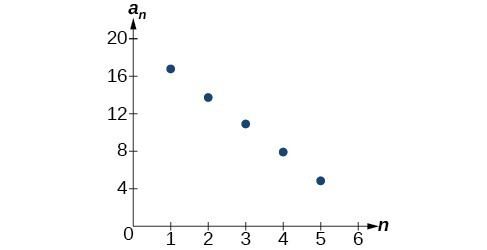Figure 2

### Try It 3

List the first five terms of the arithmetic sequence with ${a}_{1}=1$ and $d=5$ .

Solution

### How To: Given any the first term and any other term in an arithmetic sequence, find a given term.

1. Substitute the values given for ${a}_{1},{a}_{n},n$ into the formula ${a}_{n}={a}_{1}+\left(n - 1\right)d$ to solve for $d$.
2. Find a given term by substituting the appropriate values for ${a}_{1},n$, and $d$ into the formula ${a}_{n}={a}_{1}+\left(n - 1\right)d$.

### Example 3: Writing Terms of Arithmetic Sequences

Given ${a}_{1}=8$ and ${a}_{4}=14$ , find ${a}_{5}$ .

### Solution

The sequence can be written in terms of the initial term 8 and the common difference $d$ .

$\left\{8,8+d,8+2d,8+3d\right\}$

We know the fourth term equals 14; we know the fourth term has the form ${a}_{1}+3d=8+3d$ .

We can find the common difference $d$ .

$\begin{array}{ll}{a}_{n}={a}_{1}+\left(n - 1\right)d\hfill & \hfill \\ {a}_{4}={a}_{1}+3d\hfill & \hfill \\ {a}_{4}=8+3d\hfill & \text{Write the fourth term of the sequence in terms of } {a}_{1} \text{ and } d.\hfill \\ 14=8+3d\hfill & \text{Substitute } 14 \text{ for } {a}_{4}.\hfill \\ d=2\hfill & \text{Solve for the common difference}.\hfill \end{array}$

Find the fifth term by adding the common difference to the fourth term.

${a}_{5}={a}_{4}+2=16$

### Analysis of the Solution

Notice that the common difference is added to the first term once to find the second term, twice to find the third term, three times to find the fourth term, and so on. The tenth term could be found by adding the common difference to the first term nine times or by using the equation ${a}_{n}={a}_{1}+\left(n - 1\right)d$.

### Try It 4

Given ${a}_{3}=7$ and ${a}_{5}=17$ , find ${a}_{2}$ .

Solution